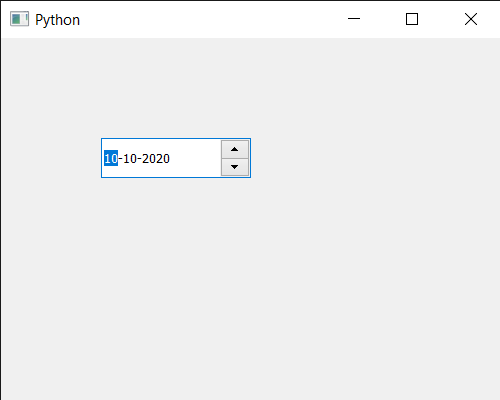GeeksforGeeks App
Open AppBrowser
Continue

# PyQt5 QDateEdit – Setting Date Time

In this article we will see how we can set date time to the QDateEdit. User can set date to the date edit with the help of cursor and the keyboard but sometimes there is a need of setting initial date or date programmatically for showing date in the records, which can be done with the help of `setDate` method. Unlike normal date, date-time has date as well as the time although time is not shown on the date edit.

In order to do this we use `setDateTime` method with the QDateEdit object

Syntax : date.setDateTime(d)

Argument : It takes QDateTime object as argument

Return : It returns None

Below is the implementation

 `# importing libraries``from` `PyQt5.QtWidgets ``import` `*` `from` `PyQt5 ``import` `QtCore, QtGui``from` `PyQt5.QtGui ``import` `*` `from` `PyQt5.QtCore ``import` `*` `import` `sys`` ` ` ` `class` `Window(QMainWindow):`` ` `    ``def` `__init__(``self``):``        ``super``().__init__()`` ` `        ``# setting title``        ``self``.setWindowTitle(``"Python "``)`` ` `        ``# setting geometry``        ``self``.setGeometry(``100``, ``100``, ``500``, ``400``)`` ` `        ``# calling method``        ``self``.UiComponents()`` ` `        ``# showing all the widgets``        ``self``.show()`` ` `    ``# method for components``    ``def` `UiComponents(``self``):`` ` `        ``# creating a QDateEdit widget``        ``date ``=` `QDateEdit(``self``)`` ` `        ``# setting geometry of the date edit``        ``date.setGeometry(``100``, ``100``, ``150``, ``40``)`` ` `        ``# date time``        ``d ``=` `QDateTime(``2020``, ``10``, ``10``, ``10``, ``20``)`` ` `        ``# setting date time``        ``date.setDateTime(d)`` ` ` ` `         ` ` ` `# create pyqt5 app``App ``=` `QApplication(sys.argv)`` ` `# create the instance of our Window``window ``=` `Window()`` ` `# start the app``sys.exit(App.``exec``())`

Output :My Personal Notes arrow_drop_up